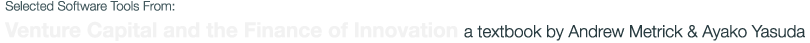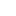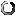# Option Calculators

The European Call Calculator lets users enter option-pricing inputs and calculates the value of a European call option using the Black-Scholes formula, as discussed in Chapter 13 of the book.

The random-expiration (European) Call Calculator implements the random-expiration version of the Black-Scholes European call formula, as discussed in Chapter 13 of the book.

The Binary Call Calculator implements the random-expiration version of the binary call formula, as discussed in Chapter 15 of the book.

## Binary Callloading application